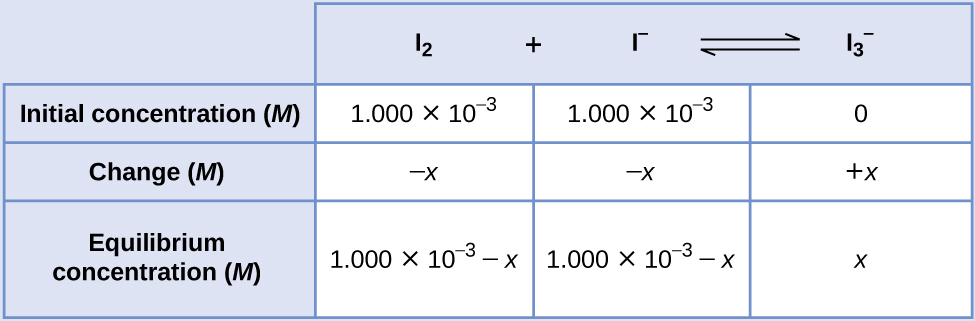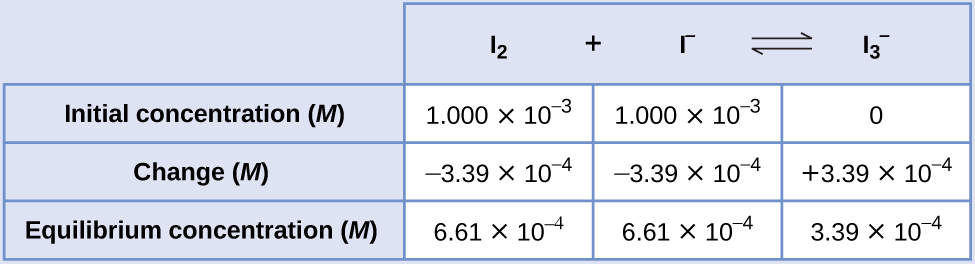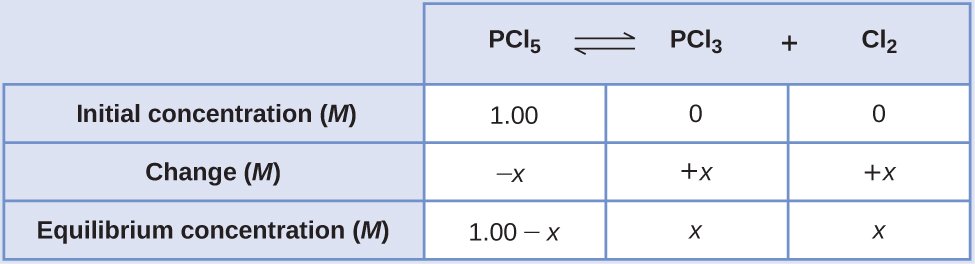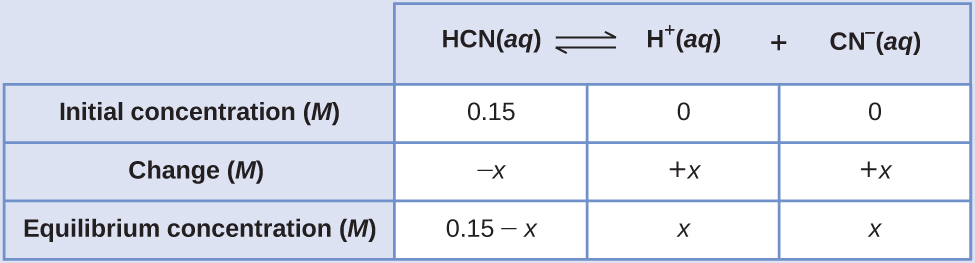17

# Equilibrium Calculations

Calculating values for equilibrium constants and/or equilibrium concentrations is of practical benefit to many applications. A mathematical strategy that uses initial concentrations, changes in concentrations, and equilibrium concentrations (and goes by the acronym ICE) is useful for performing equilibrium calculations.

## 17.1 Equilibrium Calculations

### Learning Objectives

By the end of this section, you will be able to:

• Identify the changes in concentration or pressure that occur for chemical species in equilibrium systems
• Calculate equilibrium concentrations or pressures and equilibrium constants, using various algebraic approaches
• Explain how temperature affects the spontaneity of some proceses
• Relate standard free energy changes to equilibrium constants

Having covered the essential concepts of chemical equilibria in the preceding sections of this chapter, this final section will demonstrate the more practical aspect of using these concepts and appropriate mathematical strategies to perform various equilibrium calculations. These types of computations are essential to many areas of science and technology—for example, in the formulation and dosing of pharmaceutical products. After a drug is ingested or injected, it is typically involved in several chemical equilibria that affect its ultimate concentration in the body system of interest. Knowledge of the quantitative aspects of these equilibria is required to compute a dosage amount that will solicit the desired therapeutic effect.

Many of the useful equilibrium calculations that will be demonstrated here require terms representing changes in reactant and product concentrations. These terms are derived from the stoichiometry of the reaction, as illustrated by decomposition of ammonia:

$2{\text{NH}}_{3}\left(g\right)\stackrel{}{⇌}{\text{N}}_{2}\left(g\right)+3{\text{H}}_{2}\left(g\right)$

As shown earlier in this chapter, this equilibrium may be established within a sealed container that initially contains either NH3 only, or a mixture of any two of the three chemical species involved in the equilibrium. Regardless of its initial composition, a reaction mixture will show the same relationships between changes in the concentrations of the three species involved, as dictated by the reaction stoichiometry (see also the related content on expressing reaction rates in the chapter on kinetics). For example, if the nitrogen concentration increases by an amount x:

$\text{Δ}\left[{\text{N}}_{2}\right]=+x,$

the corresponding changes in the other species concentrations are

$\begin{array}{ccc}\hfill \text{Δ}\left[{\text{H}}_{2}\right]& =\hfill & \text{Δ}\left[{\text{N}}_{2}\right]\left(\frac{3\phantom{\rule{0.2em}{0ex}}\text{mol}\phantom{\rule{0.2em}{0ex}}{\text{H}}_{\text{2}}}{1\phantom{\rule{0.2em}{0ex}}\text{mol}\phantom{\rule{0.2em}{0ex}}{\text{N}}_{\text{2}}}\right)=+3x\hfill \\ \\ \hfill \text{Δ}\left[{\text{NH}}_{3}\right]& =\hfill & -\text{Δ}\left[{\text{N}}_{2}\right]\left(\frac{2\phantom{\rule{0.2em}{0ex}}\text{mol}\phantom{\rule{0.2em}{0ex}}{\text{NH}}_{3}}{1\phantom{\rule{0.2em}{0ex}}\text{mol}\phantom{\rule{0.2em}{0ex}}{\text{N}}_{2}}\right)=-2x,\hfill \end{array}$

### EXAMPLE 17.1.3

#### Determining Relative Changes in Concentration

Derive the missing terms representing concentration changes for each of the following reactions.

(a) $\begin{array}{cccc}{\text{C}}_{2}{\text{H}}_{2}\left(g\right)+\hfill & 2{\text{Br}}_{2}\left(g\right)\hfill & ⇌\hfill & {\text{C}}_{2}{\text{H}}_{2}{\text{Br}}_{4}\left(g\right)\hfill \\ x\hfill & _____\hfill & & _____\hfill \end{array}$

(b) $\begin{array}{cccc}{\text{I}}_{2}\left(aq\right)+\hfill & {\text{I}}^{\text{−}}\left(aq\right)\hfill & ⇌\hfill & {\text{I}}_{3}{}^{\text{−}}\left(aq\right)\hfill \\ _____\hfill & _____\hfill & & x\hfill \end{array}$

(c) $\begin{array}{ccccc}{\text{C}}_{3}{\text{H}}_{8}\left(g\right)+\hfill & 5{\text{O}}_{2}\left(g\right)\hfill & ⇌\hfill & 3{\text{CO}}_{2}\left(g\right)+\hfill & 4{\text{H}}_{2}\text{O}\left(g\right)\hfill \\ x\hfill & _____\hfill & & _____\hfill & _____\hfill \end{array}$

#### Solution

(a) $\begin{array}{cccc}{\text{C}}_{2}{\text{H}}_{2}\left(g\right)+\hfill & 2{\text{Br}}_{2}\left(g\right)\hfill & ⇌\hfill & {\text{C}}_{2}{\text{H}}_{2}{\text{Br}}_{4}\left(g\right)\hfill \\ x\hfill & 2x\hfill & & -x\hfill \end{array}$

(b) $\begin{array}{cccc}{\text{I}}_{2}\left(aq\right)+\hfill & {\text{I}}^{\text{−}}\left(aq\right)\hfill & ⇌\hfill & {\text{I}}_{3}{}^{\text{−}}\left(aq\right)\hfill \\ -x\hfill & -x\hfill & & x\hfill \end{array}$

(c) $\begin{array}{lllll}{\text{C}}_{3}{\text{H}}_{8}\left(g\right)+\hfill & 5{\text{O}}_{2}\left(g\right)\hfill & ⇌\hfill & 3{\text{CO}}_{2}\left(g\right)+\hfill & 4{\text{H}}_{2}\text{O}\left(g\right)\hfill \\ x\hfill & 5x\hfill & & -3x\hfill & -4x\hfill \end{array}$

Complete the changes in concentrations for each of the following reactions:

(a) $\begin{array}{llll}2{\text{SO}}_{2}\left(g\right)+\hfill & {\text{O}}_{2}\left(g\right)\hfill & ⇌\hfill & 2{\text{SO}}_{3}\left(g\right)\hfill \\ _____\hfill & x\hfill & & _____\hfill \end{array}$

(b) $\begin{array}{lll}{\text{C}}_{4}{\text{H}}_{8}\left(g\right)\hfill & ⇌\hfill & 2{\text{C}}_{2}{\text{H}}_{4}\left(g\right)\hfill \\ _____\hfill & & -2x\hfill \end{array}$

(c) $\begin{array}{lllll}4{\text{NH}}_{3}\left(g\right)+\hfill & 7{\text{O}}_{2}\left(g\right)\hfill & ⇌\hfill & 4{\text{NO}}_{2}\left(g\right)+\hfill & 6{\text{H}}_{2}\text{O}\left(g\right)\hfill \\ \\ _____\hfill & _____\hfill & & _____\hfill & _____\hfill \end{array}$

(a) 2x, x, −2x; (b) x, −2x; (c) 4x, 7x, −4x, −6x or −4x, −7x, 4x, 6x

## 17.2 Calculation of an Equilibrium Constant

The equilibrium constant for a reaction is calculated from the equilibrium concentrations (or pressures) of its reactants and products. If these concentrations are known, the calculation simply involves their substitution into the K expression. A slightly more challenging example is provided next, in which the reaction stoichiometry is used to derive equilibrium concentrations from the information provided. The basic strategy of this computation is helpful for many types of equilibrium computations and relies on the use of terms for the reactant and product concentrations initially present, for how they change as the reaction proceeds, and for what they are when the system reaches equilibrium. The acronym ICE is commonly used to refer to this mathematical approach, and the concentrations terms are usually gathered in a tabular format called an ICE table.

### EXAMPLE 17.2.1

#### Calculation of an Equilibrium Constant

Iodine molecules react reversibly with iodide ions to produce triiodide ions.
${\text{I}}_{2}\left(aq\right)+{\text{I}}^{\text{−}}\left(aq\right)⇌{\text{I}}_{3}{}^{\text{−}}\left(aq\right)$

If a solution with the concentrations of I2 and I both equal to 1.000 $×$ 10−3 M before reaction gives an equilibrium concentration of I2 of 6.61 $×$ 10−4 M, what is the equilibrium constant for the reaction?

#### Solution

To calculate the equilibrium constants, equilibrium concentrations are needed for all the reactants and products:
$\begin{array}{ccc}{K}_{C}\hfill & =\hfill & \left[{\text{I}}_{3}{}^{\text{−}}\right]\hfill \\ \hfill & \hfill & \left[{\text{I}}_{2}\right]\left[{\text{I}}^{\text{−}}\right]\hfill \end{array}$

Provided are the initial concentrations of the reactants and the equilibrium concentration of the product. Use this information to derive terms for the equilibrium concentrations of the reactants, presenting all the information in an ICE table.At equilibrium the concentration of I2 is 6.61 $×$ 10−4 M so that

$1.000\phantom{\rule{0.2em}{0ex}}×\phantom{\rule{0.2em}{0ex}}{10}^{-3}-x=6.61\phantom{\rule{0.2em}{0ex}}×\phantom{\rule{0.2em}{0ex}}{10}^{-4}$
$x=1.000\phantom{\rule{0.2em}{0ex}}×\phantom{\rule{0.2em}{0ex}}{10}^{-3}-6.61\phantom{\rule{0.2em}{0ex}}×\phantom{\rule{0.2em}{0ex}}{10}^{-4}$
$=3.39\phantom{\rule{0.2em}{0ex}}×\phantom{\rule{0.2em}{0ex}}{10}^{-4}\phantom{\rule{0.2em}{0ex}}M$

The ICE table may now be updated with numerical values for all its concentrations:Finally, substitute the equilibrium concentrations into the K expression and solve:

${K}_{c}=\phantom{\rule{0.2em}{0ex}}\frac{\left[{\text{I}}_{3}{}^{\text{−}}\right]}{\left[{\text{I}}_{2}\right]\left[{\text{I}}^{\text{−}}\right]}$
$=\phantom{\rule{0.2em}{0ex}}\frac{3.39\phantom{\rule{0.2em}{0ex}}×\phantom{\rule{0.2em}{0ex}}{10}^{-4}\phantom{\rule{0.2em}{0ex}}M}{\left(6.61\phantom{\rule{0.2em}{0ex}}×\phantom{\rule{0.2em}{0ex}}{10}^{-4}\phantom{\rule{0.2em}{0ex}}M\right)\left(6.61\phantom{\rule{0.2em}{0ex}}×\phantom{\rule{0.2em}{0ex}}{10}^{-4}\phantom{\rule{0.2em}{0ex}}M\right)}\phantom{\rule{0.2em}{0ex}}=776$

Ethanol and acetic acid react and form water and ethyl acetate, the solvent responsible for the odor of some nail polish removers.
${\text{C}}_{2}{\text{H}}_{5}\text{OH}+{\text{CH}}_{3}{\text{CO}}_{2}\text{H}⇌{\text{CH}}_{3}{\text{CO}}_{2}{\text{C}}_{2}{\text{H}}_{5}+{\text{H}}_{2}\text{O}$

When 1 mol each of C2H5OH and CH3CO2H are allowed to react in 1 L of the solvent dioxane, equilibrium is established when $\frac{1}{3}$ mol of each of the reactants remains. Calculate the equilibrium constant for the reaction. (Note: Water is a solute in this reaction.)

Kc = 4

## 17.3 Calculation of a Missing Equilibrium Concentration

When the equilibrium constant and all but one equilibrium concentration are provided, the other equilibrium concentration(s) may be calculated. A computation of this sort is illustrated in the next example exercise.

### EXAMPLE 17.3.1

#### Calculation of a Missing Equilibrium Concentration

Nitrogen oxides are air pollutants produced by the reaction of nitrogen and oxygen at high temperatures. At 2000 °C, the value of the Kc for the reaction, ${\text{N}}_{2}\left(g\right)+{\text{O}}_{2}\left(g\right)⇌2\text{NO}\left(g\right),$ is 4.1×10−4. Calculate the equilibrium concentration of NO(g) in air at 1 atm pressure and 2000 °C. The equilibrium concentrations of N2 and O2 at this pressure and temperature are 0.036 M and 0.0089 M, respectively.

#### Solution

Substitute the provided quantities into the equilibrium constant expression and solve for [NO]:
${K}_{c}=\phantom{\rule{0.2em}{0ex}}\frac{{\left[\text{NO}\right]}^{2}}{\left[{\text{N}}_{2}\right]\phantom{\rule{0.2em}{0ex}}\left[{\text{O}}_{2}\right]}$
${\left[\text{NO}\right]}^{2}={K}_{c}\left[{\text{N}}_{2}\right]\phantom{\rule{0.2em}{0ex}}\left[{\text{O}}_{2}\right]$
$\left[\text{NO}\right]=\phantom{\rule{0.2em}{0ex}}\sqrt{{K}_{c}\left[{\text{N}}_{2}\right]\phantom{\rule{0.2em}{0ex}}\left[{\text{O}}_{2}\right]}$
$=\phantom{\rule{0.2em}{0ex}}\sqrt{\left(4.1\phantom{\rule{0.2em}{0ex}}×\phantom{\rule{0.2em}{0ex}}{10}^{-4}\right)\left(0.036\right)\left(0.0089\right)}$
$=\phantom{\rule{0.2em}{0ex}}\sqrt{1.31\phantom{\rule{0.2em}{0ex}}×\phantom{\rule{0.2em}{0ex}}{10}^{-7}}$
$=3.6\phantom{\rule{0.2em}{0ex}}×\phantom{\rule{0.2em}{0ex}}{10}^{-4}$

Thus [NO] is 3.6×10−4 mol/L at equilibrium under these conditions.

To confirm this result, it may be used along with the provided equilibrium concentrations to calculate a value for K:

$\begin{array}{ccc}\hfill {K}_{c}& =\hfill & \frac{{\left[\text{NO}\right]}^{2}}{\left[{\text{N}}_{2}\right]\phantom{\rule{0.2em}{0ex}}\left[{\text{O}}_{2}\right]}\hfill \\ & =\hfill & \frac{{\left(3.6\phantom{\rule{0.2em}{0ex}}×\phantom{\rule{0.2em}{0ex}}{10}^{-4}\right)}^{2}}{\left(0.036\right)\left(0.0089\right)}\hfill \\ & =\hfill & 4.0\phantom{\rule{0.2em}{0ex}}×\phantom{\rule{0.2em}{0ex}}{10}^{-4}\hfill \end{array}$

This result is consistent with the provided value for K within nominal uncertainty, differing by just 1 in the least significant digit’s place.

The equilibrium constant Kc for the reaction of nitrogen and hydrogen to produce ammonia at a certain temperature is 6.00×10−2. Calculate the equilibrium concentration of ammonia if the equilibrium concentrations of nitrogen and hydrogen are 4.26 M and 2.09 M, respectively.

1.53 mol/L

## 17.4 Calculation of Equilibrium Concentrations from Initial Concentrations

Perhaps the most challenging type of equilibrium calculation can be one in which equilibrium concentrations are derived from initial concentrations and an equilibrium constant. For these calculations, a four-step approach is typically useful:

1. Identify the direction in which the reaction will proceed to reach equilibrium.
2. Develop an ICE table.
3. Calculate the concentration changes and, subsequently, the equilibrium concentrations.
4. Confirm the calculated equilibrium concentrations.

The last two example exercises of this chapter demonstrate the application of this strategy.

### EXAMPLE 17.4.1

#### Calculation of Equilibrium Concentrations

Under certain conditions, the equilibrium constant Kc for the decomposition of PCl5(g) into PCl3(g) and Cl2(g) is 0.0211. What are the equilibrium concentrations of PCl5, PCl3, and Cl2 in a mixture that initially contained only PCl5 at a concentration of 1.00 M?

#### Solution

Use the stepwise process described earlier.
1. Step 1.

Determine the direction the reaction proceeds.

The balanced equation for the decomposition of PCl5 is

${\text{PCl}}_{5}\left(g\right)⇌{\text{PCl}}_{3}\left(g\right)+{\text{Cl}}_{2}\left(g\right)$

Because only the reactant is present initially Qc = 0 and the reaction will proceed to the right.

2. Step 2.

Develop an ICE table.3. Step 3.

Solve for the change and the equilibrium concentrations.

Substituting the equilibrium concentrations into the equilibrium constant equation gives

${K}_{c}=\phantom{\rule{0.2em}{0ex}}\frac{\left[{\text{PCl}}_{3}\right]\left[{\text{Cl}}_{2}\right]}{\left[{\text{PCl}}_{5}\right]}\phantom{\rule{0.2em}{0ex}}=0.0211$
$=\phantom{\rule{0.2em}{0ex}}\frac{\left(x\right)\left(x\right)}{\left(1.00-x\right)}$
$0.0211=\phantom{\rule{0.2em}{0ex}}\frac{\left(x\right)\left(x\right)}{\left(1.00-x\right)}$
$0.0211\left(1.00-x\right)={x}^{2}$
${x}^{2}+0.0211x-0.0211=0$

Appendix B shows an equation of the form ax2 + bx + c = 0 can be rearranged to solve for x:

$x=\phantom{\rule{0.2em}{0ex}}\frac{-b\phantom{\rule{0.2em}{0ex}}±\phantom{\rule{0.2em}{0ex}}\sqrt{{b}^{2}-4ac}}{2a}$

In this case, a = 1, b = 0.0211, and c = −0.0211. Substituting the appropriate values for a, b, and c yields:

$x=\phantom{\rule{0.2em}{0ex}}\frac{-0.0211\phantom{\rule{0.2em}{0ex}}±\phantom{\rule{0.2em}{0ex}}\sqrt{{\left(0.0211\right)}^{2}-4\left(1\right)\left(-0.0211\right)}}{2\left(1\right)}$
$=\phantom{\rule{0.2em}{0ex}}\frac{-0.0211\phantom{\rule{0.2em}{0ex}}±\phantom{\rule{0.2em}{0ex}}\sqrt{\left(4.45\phantom{\rule{0.2em}{0ex}}×\phantom{\rule{0.2em}{0ex}}{10}^{-4}\right)+\left(8.44\phantom{\rule{0.2em}{0ex}}×\phantom{\rule{0.2em}{0ex}}{10}^{-2}\right)}}{2}$
$=\phantom{\rule{0.2em}{0ex}}\frac{-0.0211\phantom{\rule{0.2em}{0ex}}±\phantom{\rule{0.2em}{0ex}}0.291}{2}$

The two roots of the quadratic are, therefore,

$x=\phantom{\rule{0.2em}{0ex}}\frac{-0.0211+0.291}{2}\phantom{\rule{0.2em}{0ex}}=0.135$

and

$x=\phantom{\rule{0.2em}{0ex}}\frac{-0.0211-0.291}{2}\phantom{\rule{0.2em}{0ex}}=-0.156$

For this scenario, only the positive root is physically meaningful (concentrations are either zero or positive), and so x = 0.135 M.

The equilibrium concentrations are

$\left[{\text{PCl}}_{5}\right]\phantom{\rule{0.2em}{0ex}}=\phantom{\rule{0.2em}{0ex}}1.00-0.135=0.87\phantom{\rule{0.2em}{0ex}}M$
$\left[{\text{PCl}}_{3}\right]=x=0.135\phantom{\rule{0.2em}{0ex}}M$
$\left[{\text{Cl}}_{2}\right]=x=0.135\phantom{\rule{0.2em}{0ex}}M$
4. Step 4.

Confirm the calculated equilibrium concentrations.

Substitution into the expression for Kc (to check the calculation) gives

${K}_{c}=\phantom{\rule{0.2em}{0ex}}\frac{\left[{\text{PCl}}_{3}\right]\left[{\text{Cl}}_{2}\right]}{\left[{\text{PCl}}_{5}\right]}=\phantom{\rule{0.2em}{0ex}}\frac{\left(0.135\right)\left(0.135\right)}{0.87}\phantom{\rule{0.2em}{0ex}}=0.021$

The equilibrium constant calculated from the equilibrium concentrations is equal to the value of Kc given in the problem (when rounded to the proper number of significant figures).

Acetic acid, CH3CO2H, reacts with ethanol, C2H5OH, to form water and ethyl acetate, CH3CO2C2H5.
${\text{CH}}_{3}{\text{CO}}_{2}\text{H}+{\text{C}}_{2}{\text{H}}_{5}\text{OH}⇌{\text{CH}}_{3}{\text{CO}}_{2}{\text{C}}_{2}{\text{H}}_{5}+{\text{H}}_{2}\text{O}$

The equilibrium constant for this reaction with dioxane as a solvent is 4.0. What are the equilibrium concentrations for a mixture that is initially 0.15 M in CH3CO2H, 0.15 M in C2H5OH, 0.40 M in CH3CO2C2H5, and 0.40 M in H2O?

[CH3CO2H] = 0.18 M, [C2H5OH] = 0.18 M, [CH3CO2C2H5] = 0.37 M, [H2O] = 0.37 M

A 1.00-L flask is filled with 1.00 moles of H2 and 2.00 moles of I2. The value of the equilibrium constant for the reaction of hydrogen and iodine reacting to form hydrogen iodide is 50.5 under the given conditions. What are the equilibrium concentrations of H2, I2, and HI in moles/L?
${\text{H}}_{2}\left(g\right)+{\text{I}}_{2}\left(g\right)⇌2\text{HI}\left(g\right)$

[H2] = 0.06 M, [I2] = 1.06 M, [HI] = 1.88 M

### EXAMPLE 17.4.2

#### Calculation of Equilibrium Concentrations Using an Algebra-Simplifying Assumption

What are the concentrations at equilibrium of a 0.15 M solution of HCN?
$\text{HCN}\left(aq\right)\phantom{\rule{0.2em}{0ex}}⇌\phantom{\rule{0.2em}{0ex}}{\text{H}}^{\text{+}}\left(aq\right)+{\text{CN}}^{\text{−}}\left(aq\right)\phantom{\rule{5em}{0ex}}{K}_{c}=4.9\phantom{\rule{0.2em}{0ex}}×\phantom{\rule{0.2em}{0ex}}{10}^{\text{−10}}$

#### Solution

Using “x” to represent the concentration of each product at equilibrium gives this ICE table.Substitute the equilibrium concentration terms into the Kc expression

${K}_{c}=\frac{\left(x\right)\left(x\right)}{0.15-x}$

Rearrange to the quadratic form and solve for x

${x}^{2}+4.9\phantom{\rule{0.2em}{0ex}}×\phantom{\rule{0.2em}{0ex}}{10}^{\text{−10}}-7.35\phantom{\rule{0.2em}{0ex}}×\phantom{\rule{0.2em}{0ex}}{10}^{\text{−11}}=0$
$x=8.56\phantom{\rule{0.2em}{0ex}}×\phantom{\rule{0.2em}{0ex}}{10}^{\text{−6}}\phantom{\rule{0.2em}{0ex}}M\phantom{\rule{0.2em}{0ex}}\left(\text{3 sig. figs.}\right)=8.6\phantom{\rule{0.2em}{0ex}}×\phantom{\rule{0.2em}{0ex}}{10}^{\text{−6}}\phantom{\rule{0.2em}{0ex}}M\phantom{\rule{0.2em}{0ex}}\left(\text{2 sig. figs.}\right)$

Thus [H+] = [CN] = x = 8.6×10–6 M and [HCN] = 0.15 – x = 0.15 M.

Note in this case that the change in concentration is significantly less than the initial concentration (a consequence of the small K), and so the initial concentration experiences a negligible change:

$\text{if}\phantom{\rule{0.2em}{0ex}}x\ll 0.15\phantom{\rule{0.2em}{0ex}}\text{M},\phantom{\rule{0.2em}{0ex}}\text{then}\phantom{\rule{0.2em}{0ex}}\left(0.15-x\right)\approx 0.15$

This approximation allows for a more expedient mathematical approach to the calculation that avoids the need to solve for the roots of a quadratic equation:

${K}_{c}=\phantom{\rule{0.2em}{0ex}}\frac{\left(x\right)\left(x\right)}{0.15-x}\phantom{\rule{0.2em}{0ex}}\approx \phantom{\rule{0.2em}{0ex}}\frac{{x}^{2}}{0.15}$
$4.9\phantom{\rule{0.2em}{0ex}}×\phantom{\rule{0.2em}{0ex}}{10}^{\text{−10}}=\phantom{\rule{0.2em}{0ex}}\frac{{x}^{2}}{0.15}$
${x}^{2}=\left(0.15\right)\left(4.9\phantom{\rule{0.2em}{0ex}}×\phantom{\rule{0.2em}{0ex}}{10}^{\text{−10}}\right)=7.4\phantom{\rule{0.2em}{0ex}}×\phantom{\rule{0.2em}{0ex}}{10}^{\text{−11}}$
$x=\sqrt{7.4\phantom{\rule{0.2em}{0ex}}×\phantom{\rule{0.2em}{0ex}}{10}^{\text{−11}}}=8.6\phantom{\rule{0.2em}{0ex}}×\phantom{\rule{0.2em}{0ex}}{10}^{\text{−6}}\phantom{\rule{0.2em}{0ex}}M$

The value of x calculated is, indeed, much less than the initial concentration

$8.6\phantom{\rule{0.2em}{0ex}}×\phantom{\rule{0.2em}{0ex}}{10}^{-6}\ll 0.15$

and so the approximation was justified. If this simplified approach were to yield a value for x that did not justify the approximation, the calculation would need to be repeated without making the approximation.

${\text{NH}}_{3}\left(aq\right)+{\text{H}}_{2}\text{O}\left(l\right)\phantom{\rule{0.2em}{0ex}}⇌\phantom{\rule{0.2em}{0ex}}{\text{NH}}_{4}{}^{\text{+}}\left(aq\right)+{\text{OH}}^{\text{−}}\left(aq\right)\phantom{\rule{5em}{0ex}}{K}_{\text{c}}=1.8\phantom{\rule{0.2em}{0ex}}×\phantom{\rule{0.2em}{0ex}}{10}^{\text{−5}}$
$\left[{\text{OH}}^{\text{−}}\right]=\left[{\text{NH}}_{4}{}^{\text{+}}\right]=0.0021\phantom{\rule{0.2em}{0ex}}M;$ [NH3] = 0.25 M